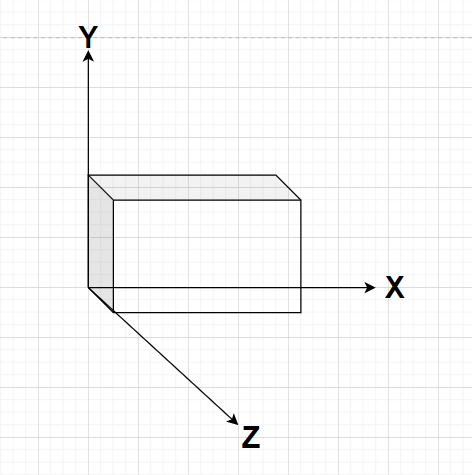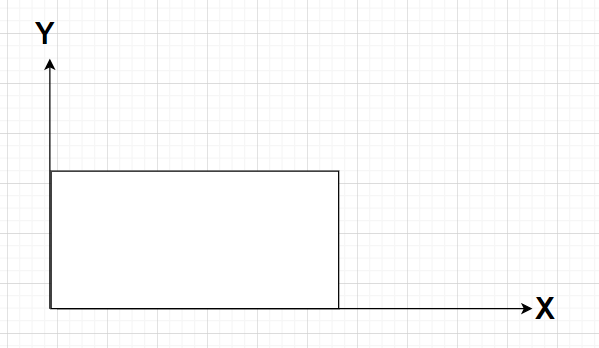Courses
Courses for Kids
Free study material
Free LIVE classes
MoreLIVE
Join Vedantu’s FREE Mastercalss

# What is the amount of surface enclosed by a closed plane figure called?${\text{A}}{\text{.}}$ area${\text{B}}{\text{.}}$ perimeter${\text{C}}{\text{.}}$ volume${\text{D}}{\text{.}}$ none of the aboveVerified
362.1k+ views
Hint- Here, we will be proceeding by understanding that actually a closed plane figure means a two dimensional figure and then defining all the terms mentioned in the options.Any plane figure means that the figure is a two dimensional figure in x-y or y-z or x-z plane i.e., the third coordinate is always zero.
For example: In the above figures, the rectangle is a closed plane figure (two dimensional figure) whose z coordinate is zero (only x and y coordinates exist) whereas the cube in the other figure is a closed three dimensional figure.

Area refers to the amount of surface enclosed by a closed two dimensional figure. So, the area is called the amount of surface enclosed by a closed plane figure.
Perimeter refers to the amount of the boundary of any surface enclosed by a closed plane figure or a closed two dimensional figure. So, the perimeter is not called the amount of surface enclosed by a closed plane figure.
Volume refers to the three dimensional space enclosed by a boundary or occupied by an object. So, volume is not called the amount of surface enclosed by a closed plane figure.
So, for the rectangle given in the figure area and perimeter exists whereas volume doesn’t exist and for the cube given, volume exists.

Clearly, option A is correct.

Note- In these types of problems, we know that area and perimeter are always associated with a closed two-dimensional figure or closed plane figure whereas volume is always associated with a closed three dimensional space which is enclosed by a boundary or by an object.
Last updated date: 24th Sep 2023
Total views: 362.1k
Views today: 11.62k